or# RD Sharma Class 10 and Chapter Areas Related To Circles Maths Solutions

## Maths Class 10 and Chapter Areas Related To Circles RD Sharma SolutionsFilter
Filters :
CLASSES
•
•
•
•
•
•
•
CHAPTERS
•
• 6 More

### RD SHARMA Class 10 | AREAS RELATED TO CIRCLES

Find to the three places of decimal the radius of the circle whose area is the sum of the areas of two triangles whose sides are 35, 53, 66 and 33, 56, 65 measured in centimetres.We have learned about Coordinate Geometry in our previous chapter.  Here, in this chapter, we will learn about Areas related to Circles. You would be able to understand the right idea on how to find the length of the arc. Geometry is such subject where it requires the perfect knowledge on how to come up with the right solution. Doubtnut has provided the students with lots exercises and examples which helps them to get the best idea on how to work on the different problems.

It has been seen that students who follow NCERT textbooks hardly scores good marks in the exams. This is because these textbooks fail to provide the in-depth knowledge that makes students remain ignorant. So, with the hope of achieving success in the exams, students try to opt for the different reference books or guides. Class 10 Maths RD Sharma Solutions proves to be of much help to the students where they get the best idea on how to attempt different questions. Still students look for the best reference books so as to score high marks in the exams.

With the aim to make students get the right idea on how to attempt even the trickiest questions, a team of experienced tutors have designed an online study material. Students are able to clear their queries without any sort of problem at all. These study materials are free for them to access. The best thing is that it can be accessed from any place no matter where you are. Thus, with the use of the best video tutorials, it does not lead them to stay confused at all. So, students who wish to excel in their exams can always look forward to finding the best solutions in the perfect manner.

## Topics and subtopics of RD Sharma class 10 Chapter 15 Areas Related to Circles

### Finding the length of the arc

It is possible to find the length of the arc in the best way. Let us have a look at some of the exercises.

Exercise 1:   Find the length of the arc that subtends an angle of 30 degrees in terms of π, at the center of O of the circle with a radius of 4 cm.

Solution:

Given Data:

Angle subtended at the centre 'O' = 30°

Formula to be used: Length of arc = θ/360 × 2πr cm

Length of arc = 30/360 × 2π4 cm = 2π/3 cm

Therefore, the length of the arc that subtends an angle of 60 degrees is 2π/3cm

Exercise 2: With a length 12 cm a chord PQ of subtends an angle 120 at the center of a circle.  Can you calculate the area of the minor segment cut off by the chord PQ?

Solution:

We know that, Area of the segment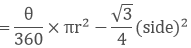We have, /POQ = 120 and PQ = 12cm

PL = PQ × (0.5) = 12 × 0.5 = 6 cm

Since, /POQ = 120

/POL = /QOL = 60

In triangle OPQ, we have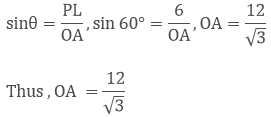With the use of the value of r and angle θ. We will find the area of minor segment.Exercise 3: The minute hand of a circle is √21 cm long. You need to calculate the area which is described by the minute hand on the face of clock between 7:00 a.m to 7:05 a.m.

Solution:

Given data: Radius of the minute hand (r) = √21cm

Time between 7: 00 a. m to 7: 05 a. m = 5 min

As we know that 1 hr = 60 min, minute hand completes

One revolution = 360° 60 min = 360°

θ = Angle subtended at the centre of circle = 5 × 6° = 30°

Area of the sector = θ/360 πr2

Area of the sector = 30/360  pi352

Area of the sector = 5.5 cm2

Therefore, Area of the sector is 5.5 cm2

Exercise 4:  In a circle of radius 6 cm, the length of the chord is 10 cm and it makes an angle of 110° at the centre of circle. Calculate:

(i) Circumference of the circle

(ii) Area of the circle

(iii) Length of arc

(iv) The area of sector

Solution:

Here we have: Radius of the circle = 6 cm

Chord of length = 10 cm

Angle which is subtended by chord with the centre of the circle is = 110°

Now we use the formula: Circumference of a circle = 2

Area of a Circle = Area of the sector = θ/360 πr2

Length of arc = 90/360 2πx 28cm

Circumference of a circle = 2

= 2 × 3.14 × 8 = 37.7 cm

Area of a Circle = 3.14 × 6 × 6 = 113.14 cm2

Area of the sector = θ/360 πr/2

Area of the sector = 110/360 π62

On solving the above equation we get,

Area of the sector = 33.1 cm2

Length of arc = θ/360 2πr cm [latex]

Length of arc = 110/360 2π6cm

Now after solving we get, Length of arc = 22.34 cm.

Therefore, Circumference = 37.7 cm

Area of a Circle = 113.14 cm2

Area of the sector = 33.1 cm2

Exercise 5: Look at the given figure. You can find that the sector of a circle with centre 'O' subtends to an angle θ°. Prove that: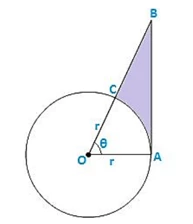1. Perimeter of shaded region is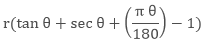2. Area of the shaded region is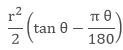Solution:

We have the data: Angle which is subtended at the centre of the circle is = θ°

Now /OAB = 90° [at point of contact, tangent is perpendicular to radius]

OAB is a right angle triangle

cos θ = adjside/hypotenuse = r/OB = OB = r sec θ

sec θ = opposite/adjside = AB/r = AB = r tan θ

Now we can find the perimeter of the shaded region = AB + BC + CA (arc)

=  r tan? + (OB - OC) + θ/360 2πr cm

= r (tan θ + sec θ + π θ/180 − 1)

Now coming to the areas of the shaded region = (Area of triangle AOB) - (Area of the sector)

(1/2 OA AB) − θ/360 πr2

On solving the above equation we get, r2/2[tan θ − πθ/180]

## Role played by Doubtnut for the students

Doubtnut has made it much easier for the students to tackle different questions in the right way. It also becomes possible for them to come up with an accurate solution. Geometry becomes much easier for them where it also makes them feel quite confident as well. Well, we will have a look at some of the advantages of Doubtnut that can be enjoyed by the students

• Provides step by step solutions: The team of Doubtnut has really made good effort in providing students with the step by step solutions. This proves to be much useful to the students who do not find any problem in coming up with the perfect answers.  This in turn helps to score good marks in the examination. Therefore students are able to develop a liking towards the subject.
• Easy and free to download: The online study materials can also be downloaded easily in the pdf format. Being free, it does not lead to spending any amount of money at all. Once it gets downloaded, students can try to access it from their own comfort. So, it proves to be quite flexible for them where they can practice the exercises without any worry at all.
• Expect the best support: It is also possible to expect the best support from the team of Doubtnut. This proves to be of much use to the students where they do not have to stay confused or ignorant at all. So, taking the best step to opt the video tutorials can truly benefit the students to pass their exams with flying colours without any problem at all.